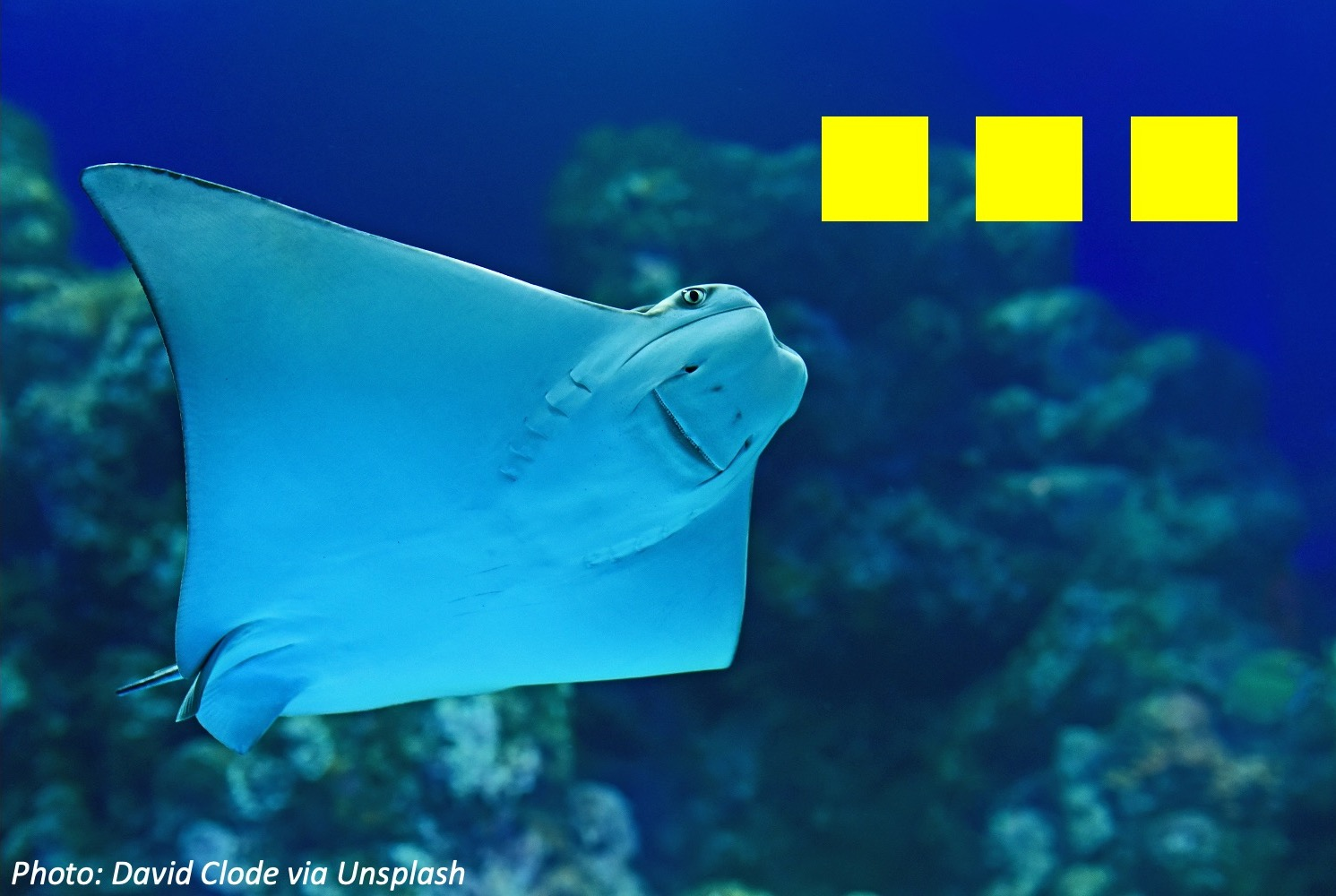# Can Fish Do Math?Can fish do math? Some scientists showed shapes, like 3 squares, to a fish. If the 3 squares were blue, that fish had to swim to a door with 1 more square on it. If the 3 squares were yellow, the fish had to subtract 1 and swim to a door with 2 squares. The fish earned snacks for right answers. Well, 6 out of 8 cichlids and 4 out of 8 stingrays were able to add and subtract! Maybe it’s not so surprising, since fish are always in schools.

Wee ones: Imagine you’re a stingray taking the test. You see 4 blue squares, so you have to swim to the door that has 1 more square than that. How many squares on the next door?

Little kids: 6 cichlids and 4 stingrays did the math right. How many more cichlids than stingrays is that? Bonus: If those 6 cichlid fish got 3 treats each, how many treats did they win?

Big kids: If 4 of 8 stingrays can do math, how many stingrays in a group of 20 would you expect to do math? Why? Bonus: Some sharks decide they want to play, too. If there are twice as many sharks as stingrays taking the quiz, and twice as many cichlids as sharks, and there are 42 creatures in total…how many of each creature are doing math?

The sky’s the limit: If there are 100 total fish in a group of cichlids and stingrays, and an equal number of cichlids and stingrays in that group can do math, how many of each type of fish must there be? (Reminder: 3/4 of cichlids and 1/2 of stingrays can do math).

Wee ones: 5.

Little kids: 2 more, because 6 – 4 = 2. Bonus: 18 treats: you can add it up 3 + 3 + 3 + 3 + 3 + 3 or 6 + 6 + 6.

Big kids: 10, because 4 is 1/2 of 8, and 20 / 2 = 10. Bonus: 6 stingrays, 12 sharks and 24 cichlids. Quick way: each stingray has 2 shark friends and 4 cichlids, which is a set of 7. And there are 6 such sets in 42. You can also write this out with algebra!

The sky’s the limit: 40 cichlids and 60 stingrays. 3/4 x 40 = 30 = 1/2 x 60. You can get this by trial and error, but using two-step algebra:

c + s = 100

3/4 c = 1/2 s

That means 1 s = 3/2 c. Substituting that into the first equation,

c + 3/2 c = 100

5/2 c = 100 (add them together)

5 c = 200 (double each side)

c = 40 cichlids, so then we have 100-40=60 stingrays.

## Pick a Topic

50 States

Animals

Daily Routine

Entertainment

Food

History

Science and Nature

Sports

Vehicles and Transportation